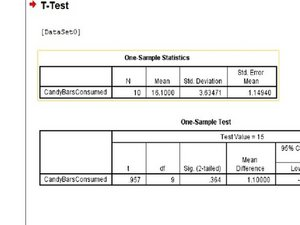Zum Hauptinhalt wechseln

## Änderungen an Schritt Nr. 12

Bearbeitet von mikaylalynnnelson -

Bearbeitung genehmigt von mikaylalynnnelson

Vorher
Nachher
Unverändert

### Schritt-Zeilen

 [title] Charts?Understanding the Results[* black] Insert wisdom hereThe first chart is the Descriptive Statistics. [* black] The first column is the number of participants. [* black] The second column is the mean. [* black] '''Mean''': the average of a set of values. [* black] The third column is the Standard Deviation. [* black] '''Standard Deviation''': a measure that is used to show how much the results vary between a set of data values. [* black] The fourth column is the standard error of the mean. [* black] '''Standard Error''': The probability that the difference between the difference in means between the control group and experimental group is greater than zero. [title] Charts?Understanding the Results[* black] Insert wisdom hereThe first chart is the Descriptive Statistics. [* black] The first column is the number of participants. [* black] The second column is the mean. [* black] '''Mean''': the average of a set of values. [* black] The third column is the Standard Deviation. [* black] '''Standard Deviation''': a measure that is used to show how much the results vary between a set of data values. [* black] The fourth column is the standard error of the mean. [* black] '''Standard Error''': The probability that the difference between the difference in means between the control group and experimental group is greater than zero.

Bild 1

Kein vorheriges BildHinzugefügt